# RD Sharma Solutions For Class 12 Maths Exercise 4.8 Chapter 4 Inverse Trigonometric Functions

RD Sharma Solutions Class 12 Maths Exercise 4.8 Chapter 4 Inverse Trigonometric Functions is provided here. Exercise 4.8 of Chapter 4 deals with the properties of inverse functions. By using these properties we have to solve given problems. These concepts are explained in the best possible way by a set of experts at BYJU’S to help students prepare effectively for the board exam. The solutions are prepared in a stepwise manner as each step carries marks in the latest CBSE exam pattern.

Students can refer to the PDF of RD Sharma Solutions while solving exercise wise problems to get their doubts cleared immediately. RD Sharma Solutions Class 12 Maths Chapter 4 Inverse Trigonometric Functions Exercise 4.8 PDF are available here.

## Download the PDF of RD Sharma Solutions For Class 12 Chapter 4 – Inverse Trigonometric Functions Exercise 4.8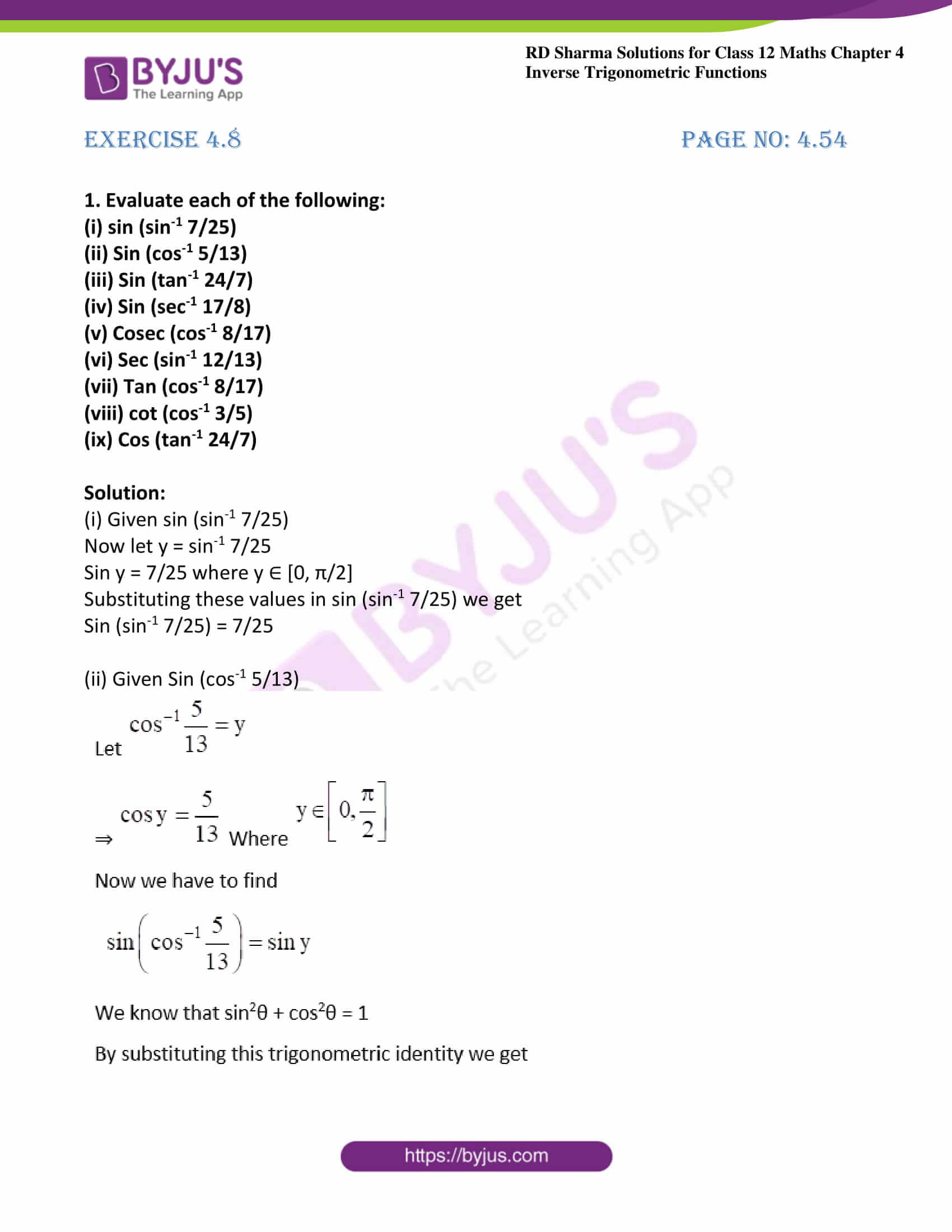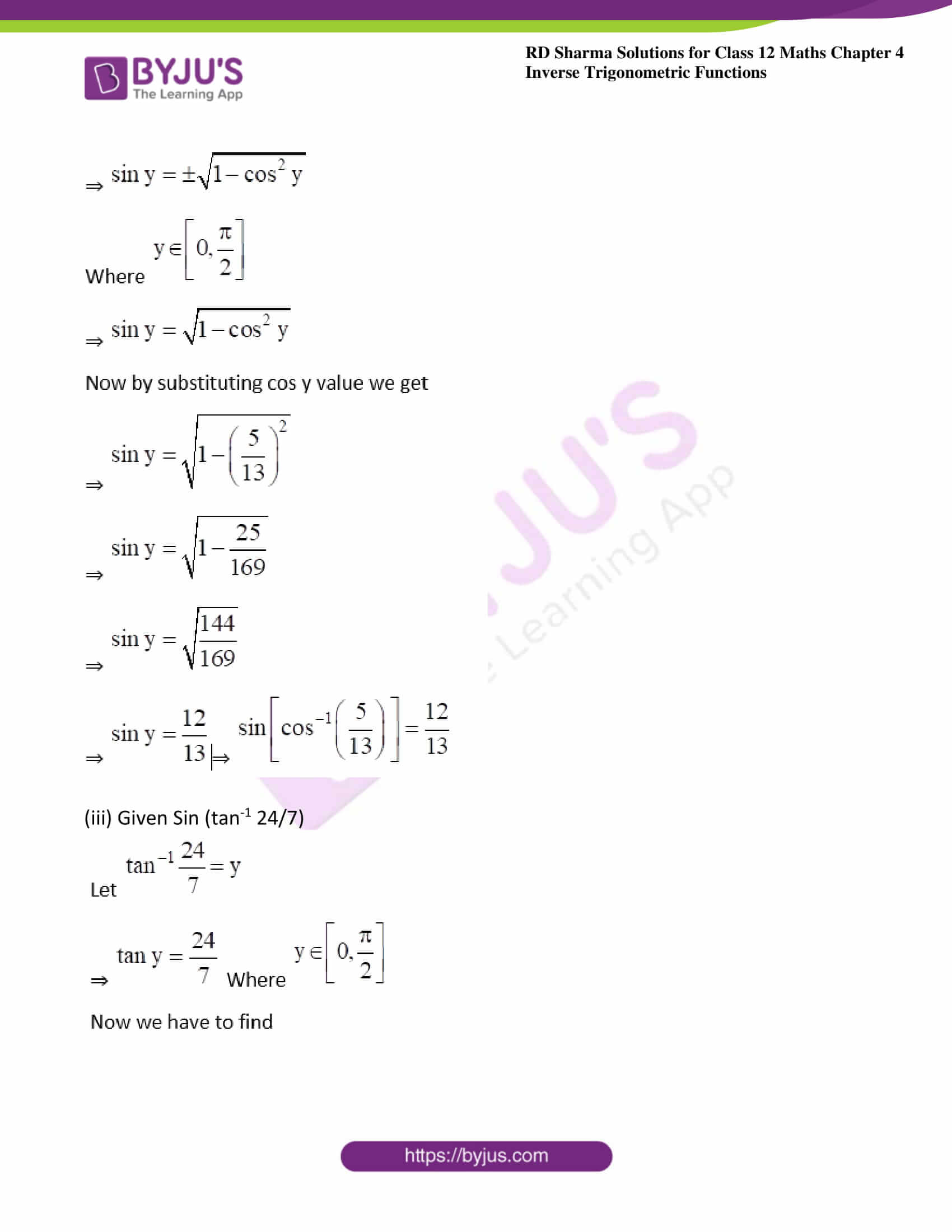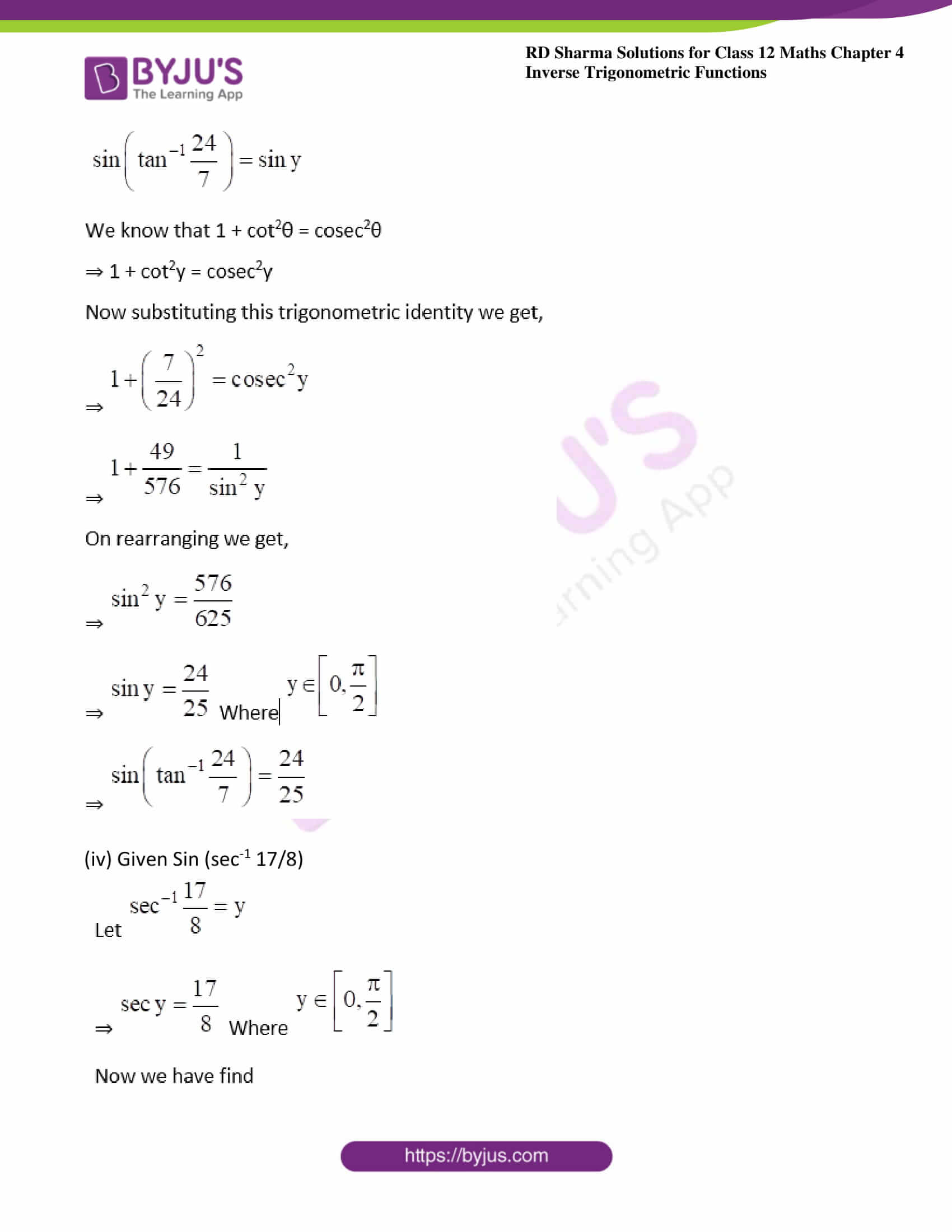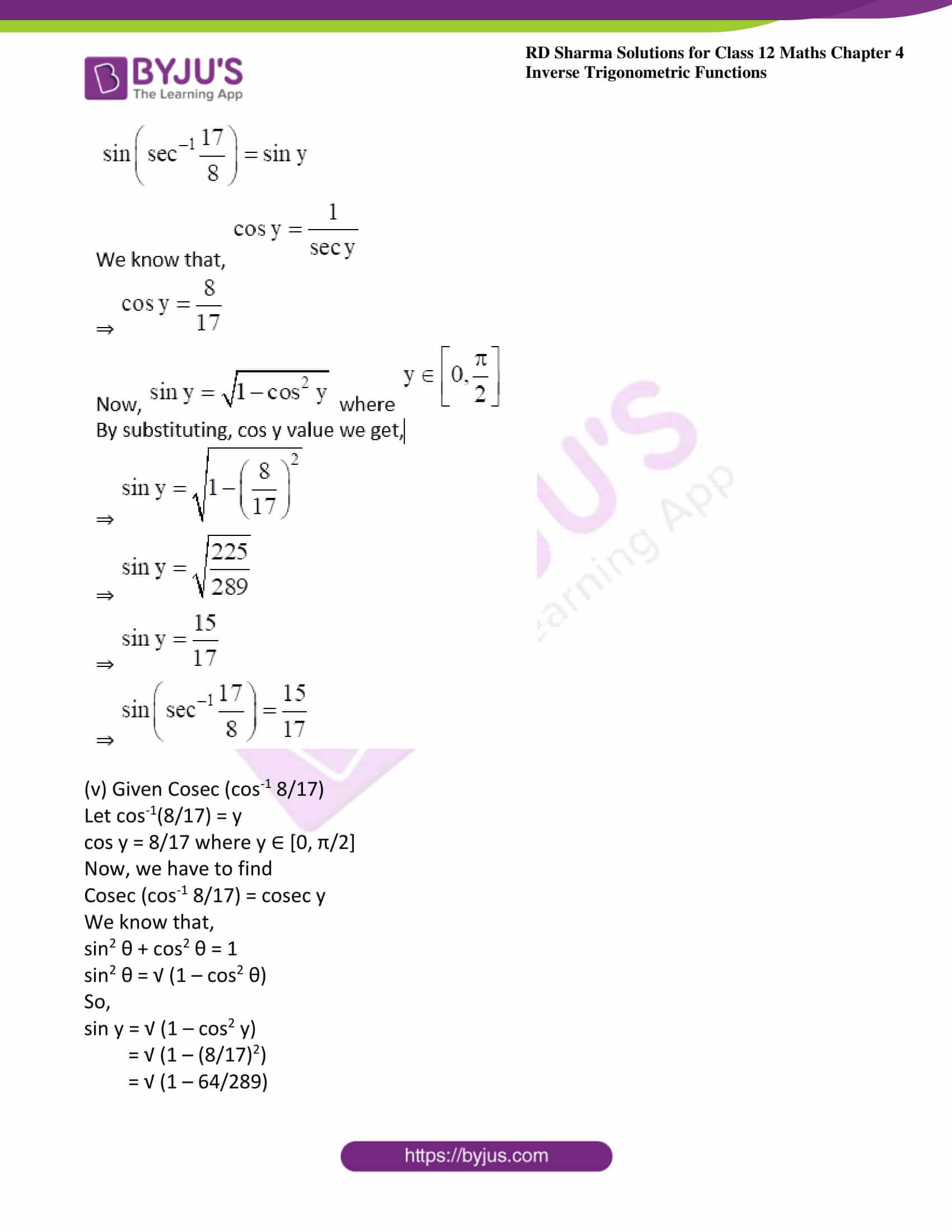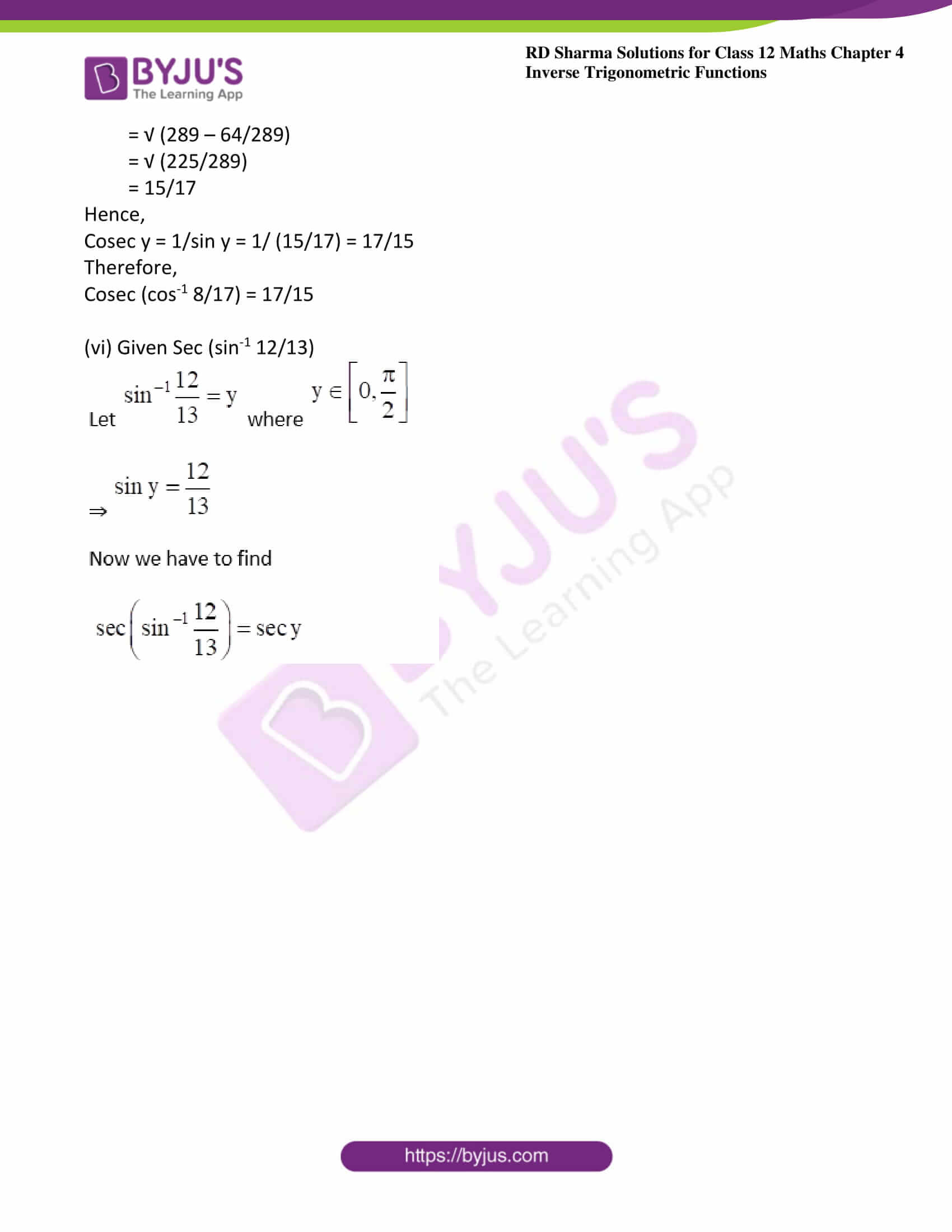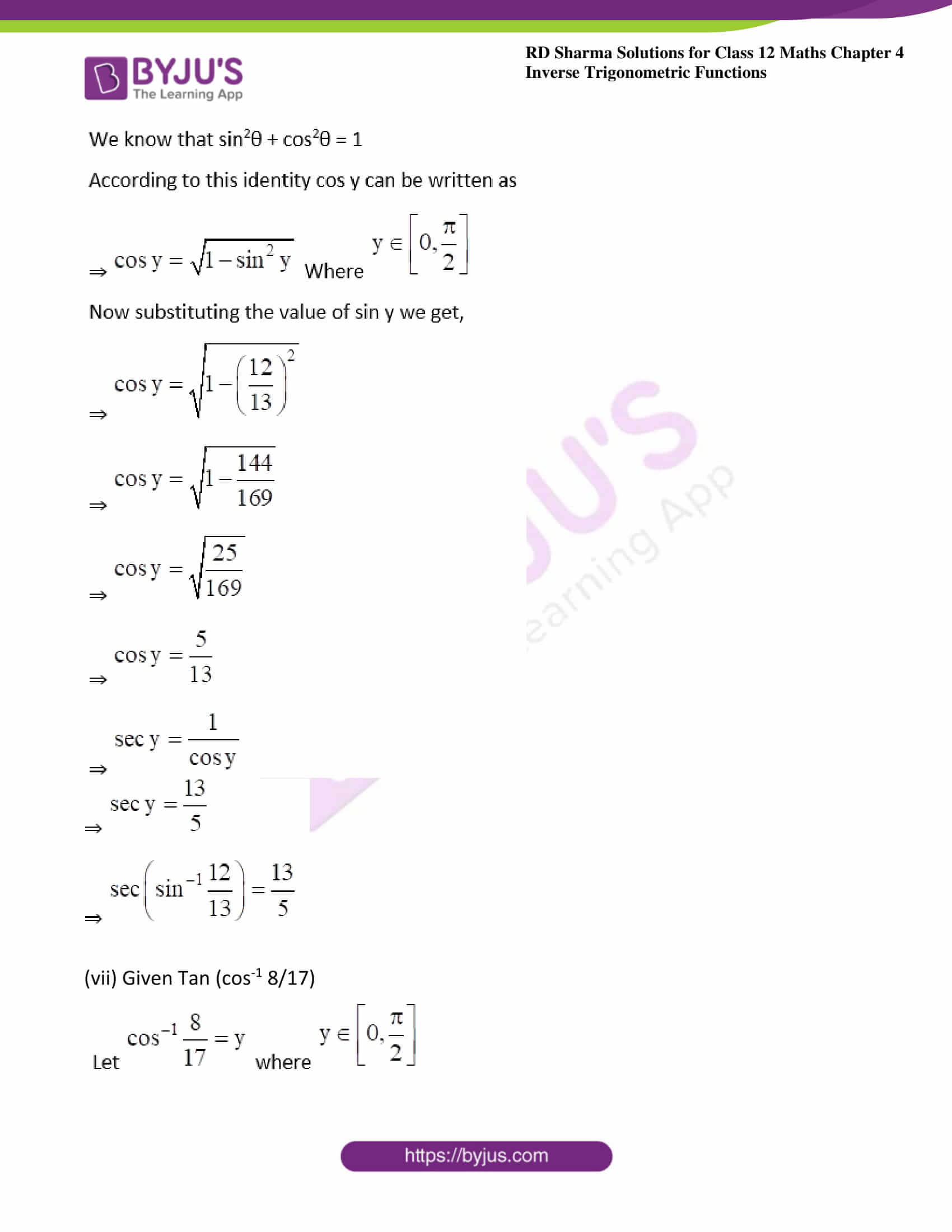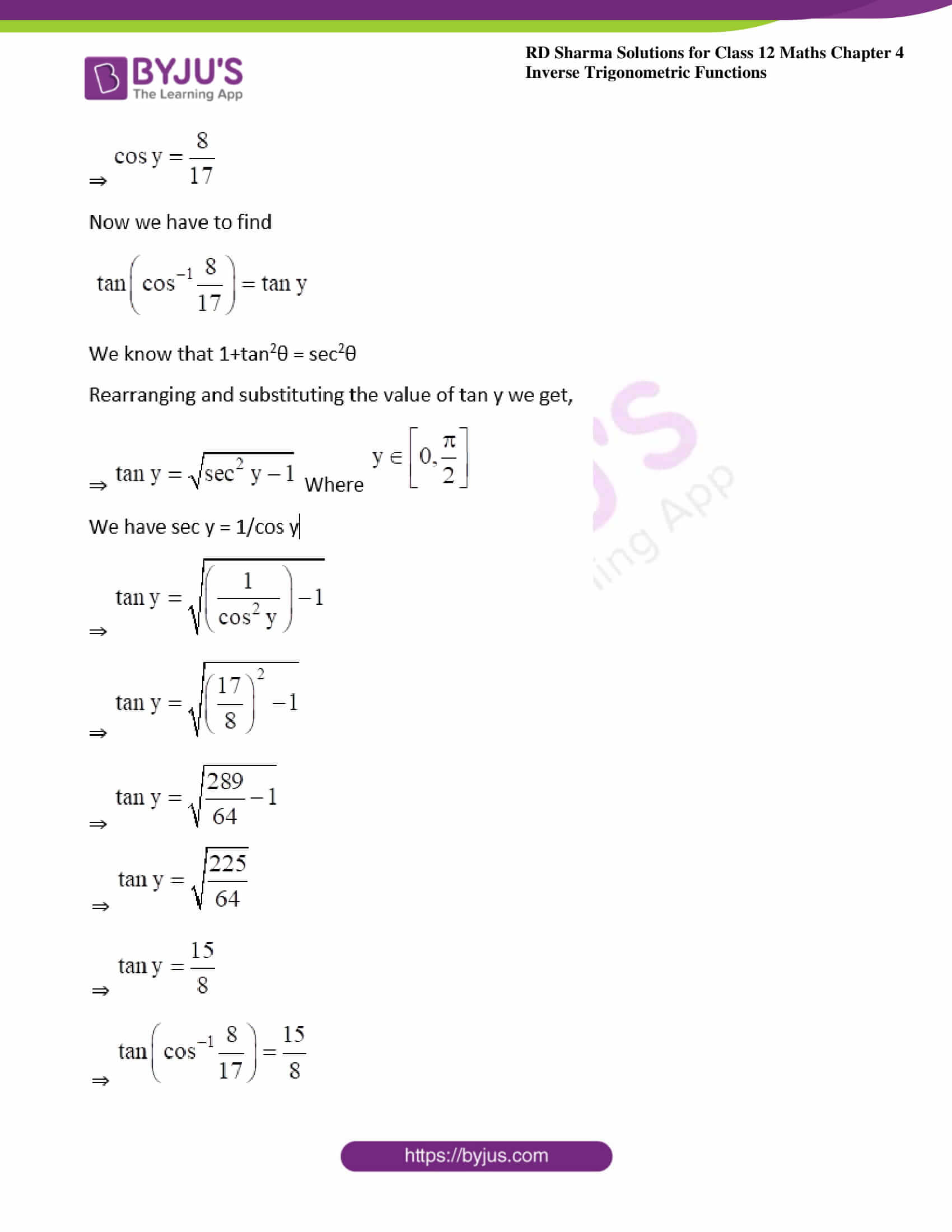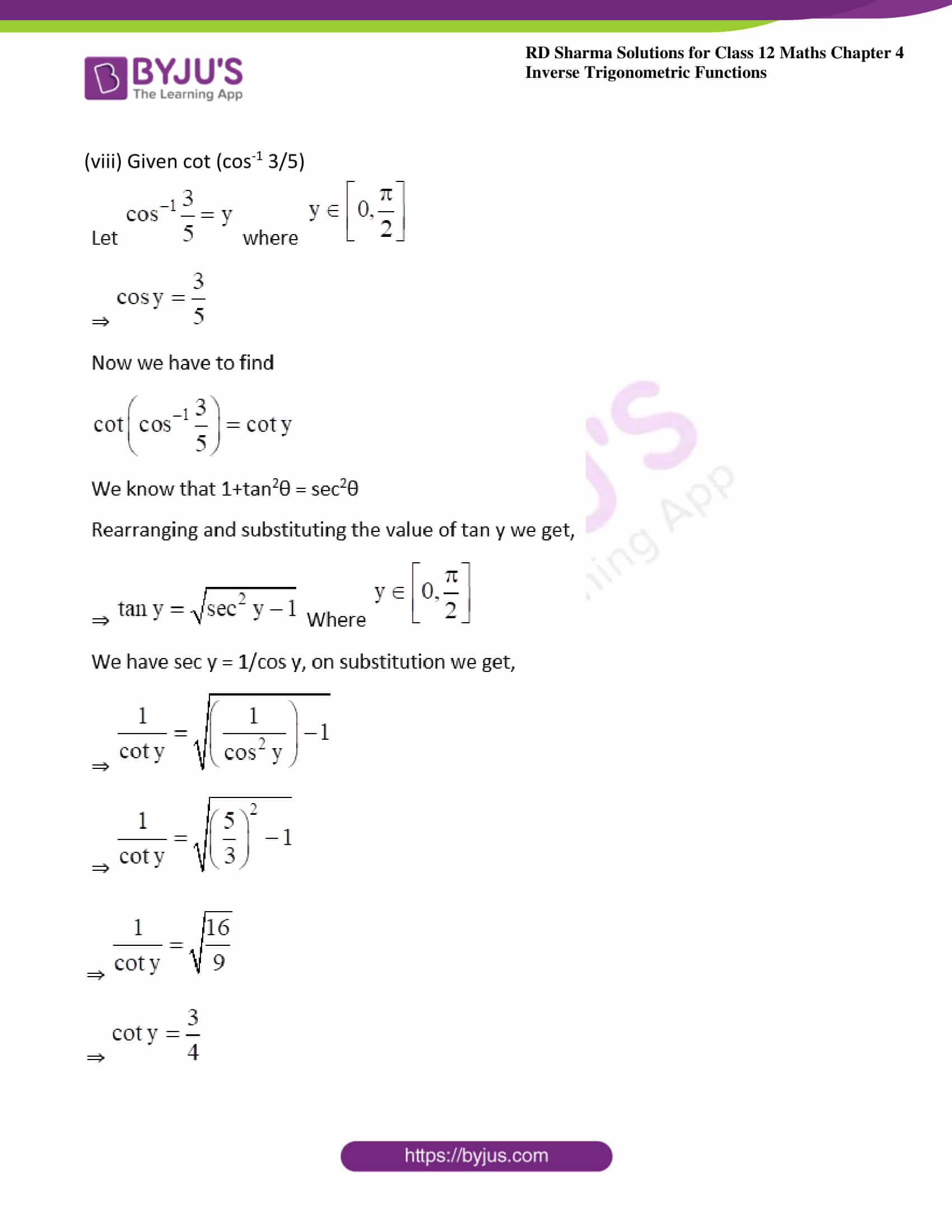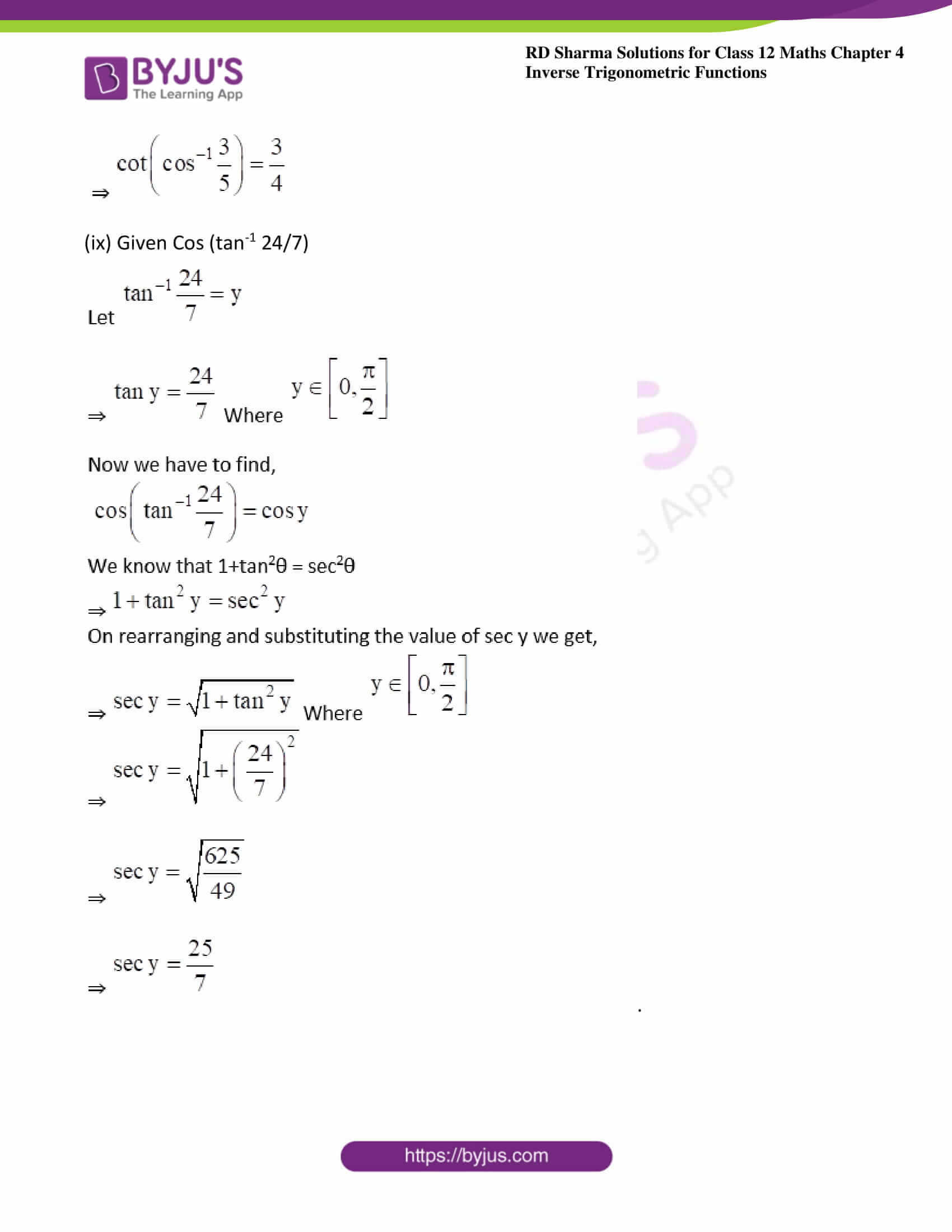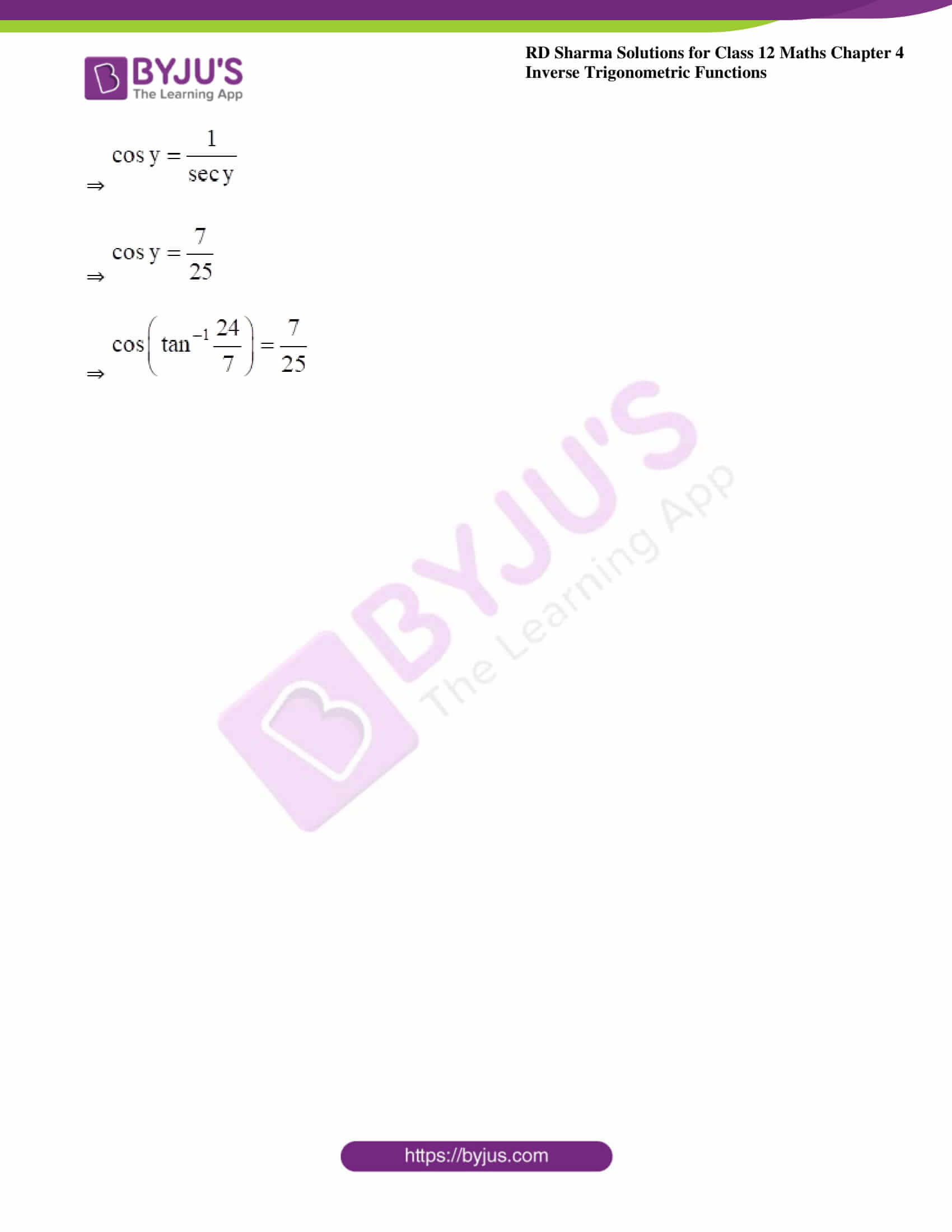### Exercise 4.8 Page No: 4.54

1. Evaluate each of the following:

(i) sin (sin-1 7/25)

(ii) Sin (cos-1 5/13)

(iv) Sin (sec-1 17/8)

(v) Cosec (cos-1 8/17)

(vi) Sec (sin-1 12/13)

(vii) Tan (cos-1 8/17)

(viii) cot (cos-1 3/5)

Solution:

(i) Given sin (sin-1 7/25)

Now let y = sin-1 7/25

Sin y = 7/25 where y ∈ [0, π/2]

Substituting these values in sin (sin-1 7/25) we get

Sin (sin-1 7/25) = 7/25

(ii) Given Sin (cos-1 5/13)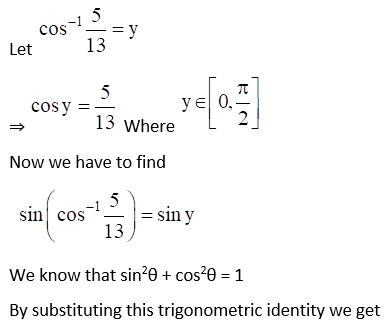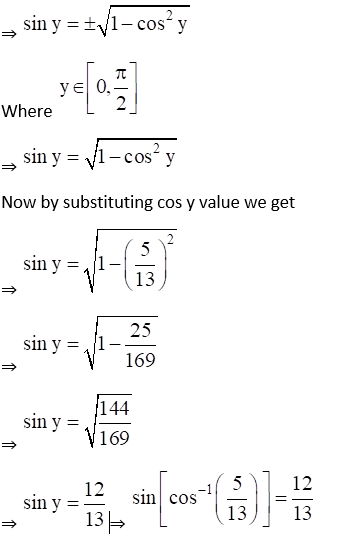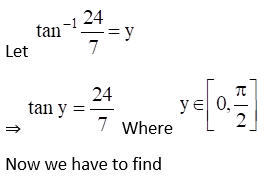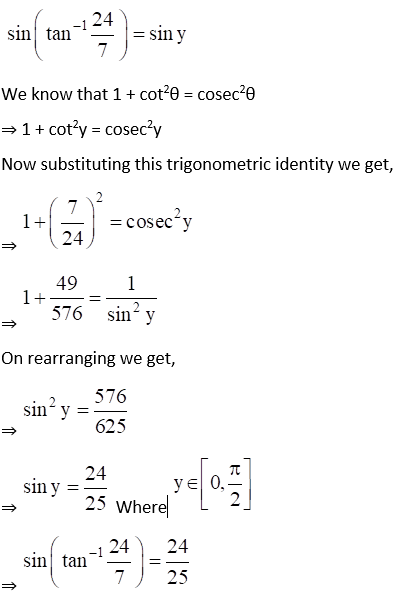(iv) Given Sin (sec-1 17/8)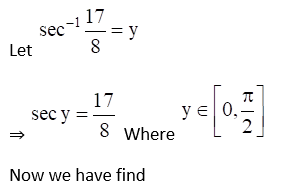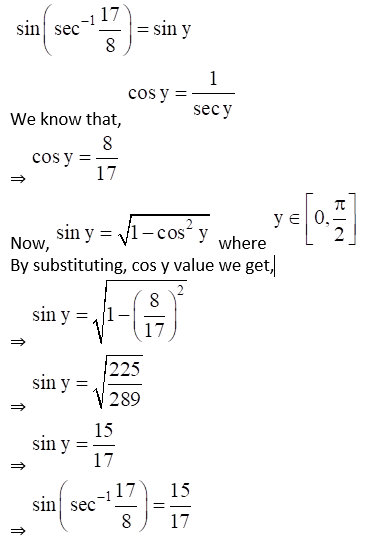(v) Given Cosec (cos-1 8/17)

Let cos-1(8/17) = y

cos y = 8/17 where y ∈ [0, π/2]

Now, we have to find

Cosec (cos-1 8/17) = cosec y

We know that,

sin2 θ + cos2 θ = 1

sin2 θ = √ (1 – cos2 θ)

So,

sin y = √ (1 – cos2 y)

= √ (1 – (8/17)2)

= √ (1 – 64/289)

= √ (289 – 64/289)

= √ (225/289)

= 15/17

Hence,

Cosec y = 1/sin y = 1/ (15/17) = 17/15

Therefore,

Cosec (cos-1 8/17) = 17/15

(vi) Given Sec (sin-1 12/13)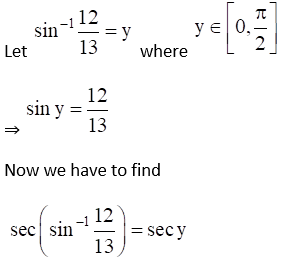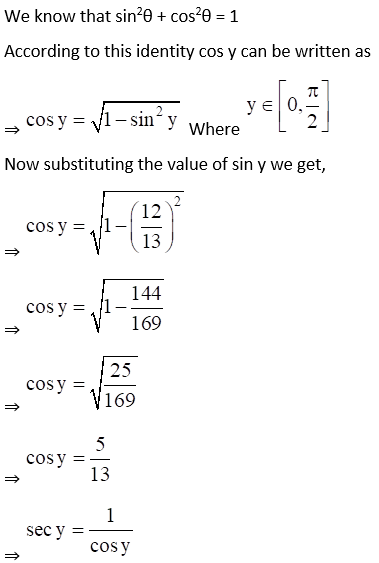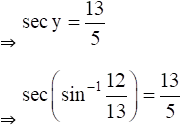(vii) Given Tan (cos-1 8/17)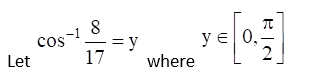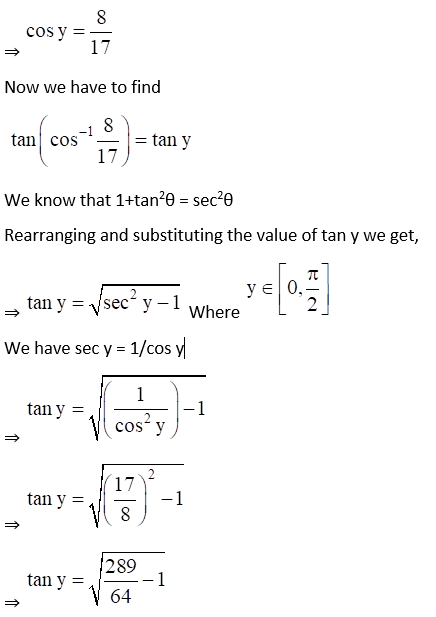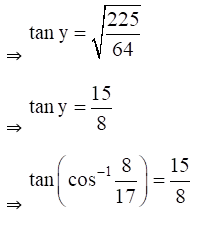(viii) Given cot (cos-1 3/5)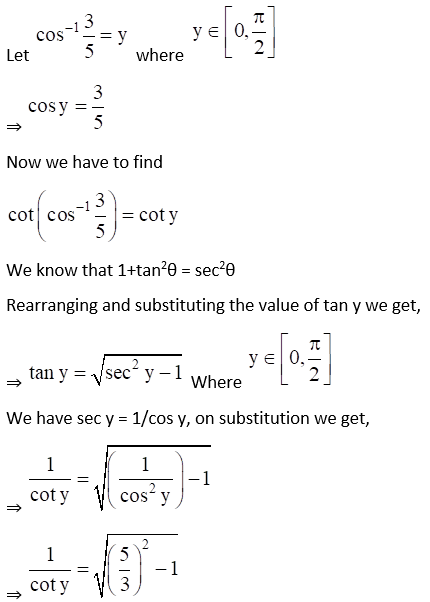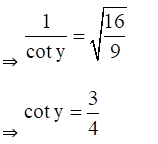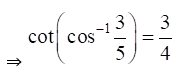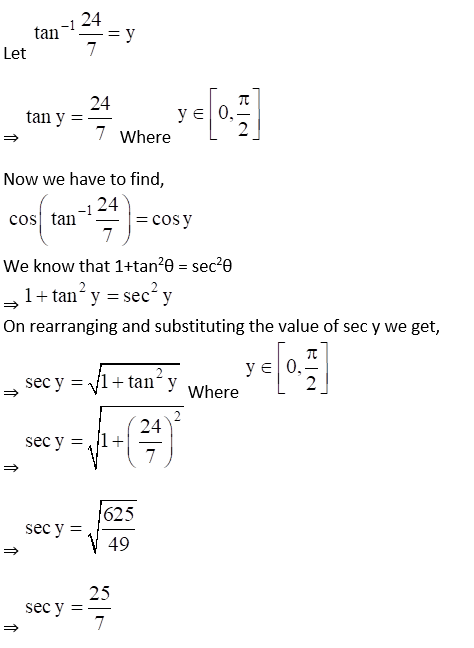.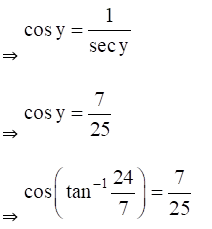### Access other exercises of RD Sharma Solutions For Class 12 Chapter 4 – Inverse Trigonometric Functions

Exercise 4.1 Solutions

Exercise 4.2 Solutions

Exercise 4.3 Solutions

Exercise 4.4 Solutions

Exercise 4.5 Solutions

Exercise 4.6 Solutions

Exercise 4.7 Solutions

Exercise 4.9 Solutions

Exercise 4.10 Solutions

Exercise 4.11 Solutions

Exercise 4.12 Solutions

Exercise 4.13 Solutions

Exercise 4.14 Solutions